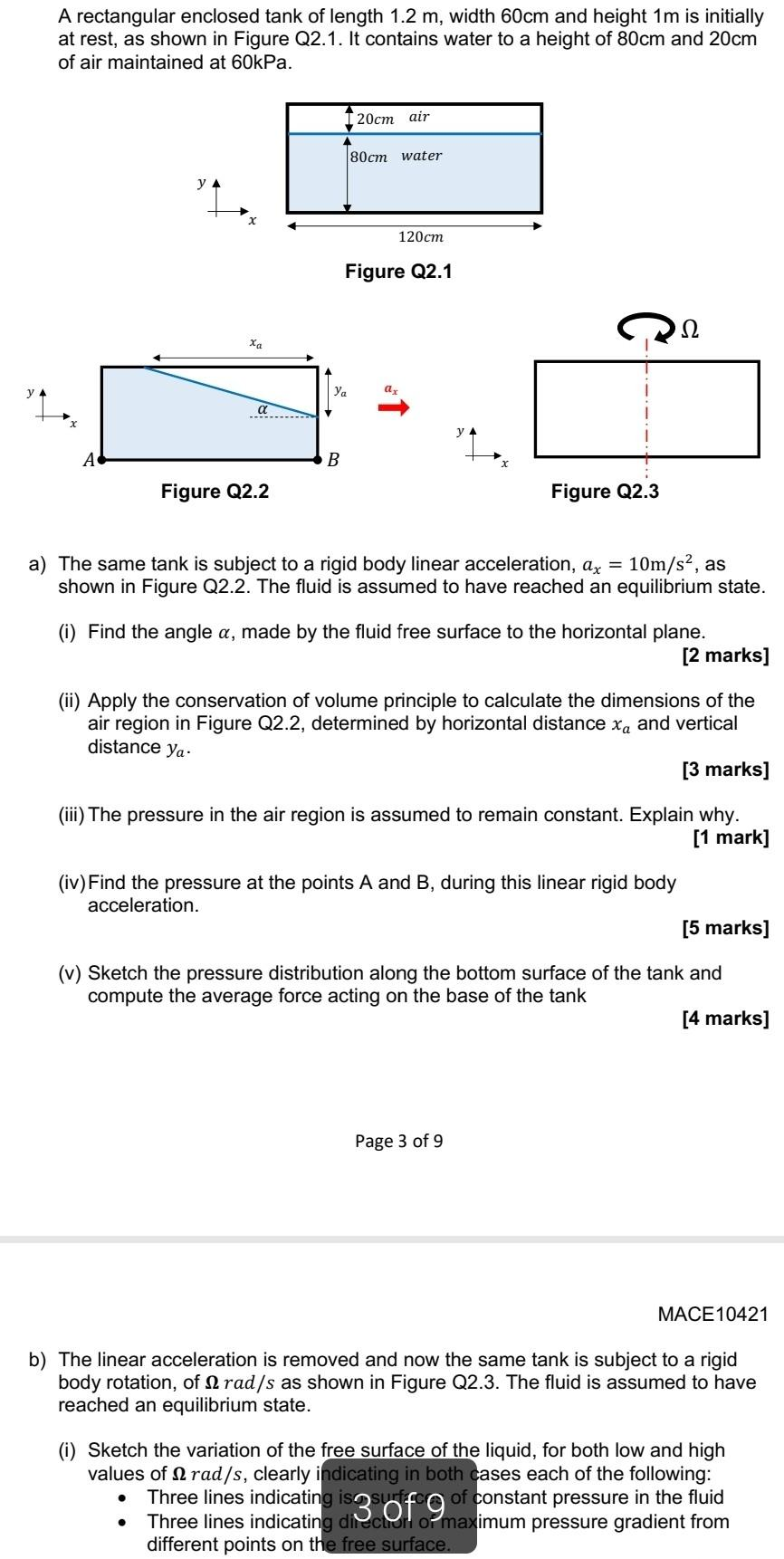Home / Expert Answers / Mechanical Engineering / a-rectangular-enclosed-tank-of-length-1-2m-width-60cm-and-height-1m-is-initially-at-rest-pa676

# (Solved): A rectangular enclosed tank of length 1.2m, width 60cm and height 1m is initially at rest ...A rectangular enclosed tank of length , width and height is initially at rest, as shown in Figure Q2.1. It contains water to a height of and of air maintained at . a) The same tank is subject to a rigid body linear acceleration, , as shown in Figure Q2.2. The fluid is assumed to have reached an equilibrium state. (i) Find the angle , made by the fluid free surface to the horizontal plane. [2 marks] (ii) Apply the conservation of volume principle to calculate the dimensions of the air region in Figure Q2.2, determined by horizontal distance and vertical distance . [3 marks] (iii) The pressure in the air region is assumed to remain constant. Explain why. [1 mark] (iv) Find the pressure at the points and , during this linear rigid body acceleration. [5 marks] (v) Sketch the pressure distribution along the bottom surface of the tank and compute the average force acting on the base of the tank [4 marks] Page 3 of 9 MACE10421 b) The linear acceleration is removed and now the same tank is subject to a rigid body rotation, of as shown in Figure Q2.3. The fluid is assumed to have reached an equilibrium state. (i) Sketch the variation of the free surface of the liquid, for both low and high values of , clearly i ases each of the following: - Three lines indicatin :onstant pressure in the fluid - Three lines indicatin imum pressure gradient from different points on th

We have an Answer from Expert